# Team:Shanghai Metro HS/Model

Shanghai_Metro_HSMODEL
As our goal is to produce the additive that could secret PKC enzyme to help degrade the cellulose in silage, we want to ensure that our engineered strain can make the best of itself at its best condition. From this perspective, we designed several different induction conditions for protein expression and decided to build the model to determine the optimal condition for our engineered strain to “work” better.
Below is the initial data:
Table 1. The gray value of E. coli/PKC-OP under different concentrations of IPTG against time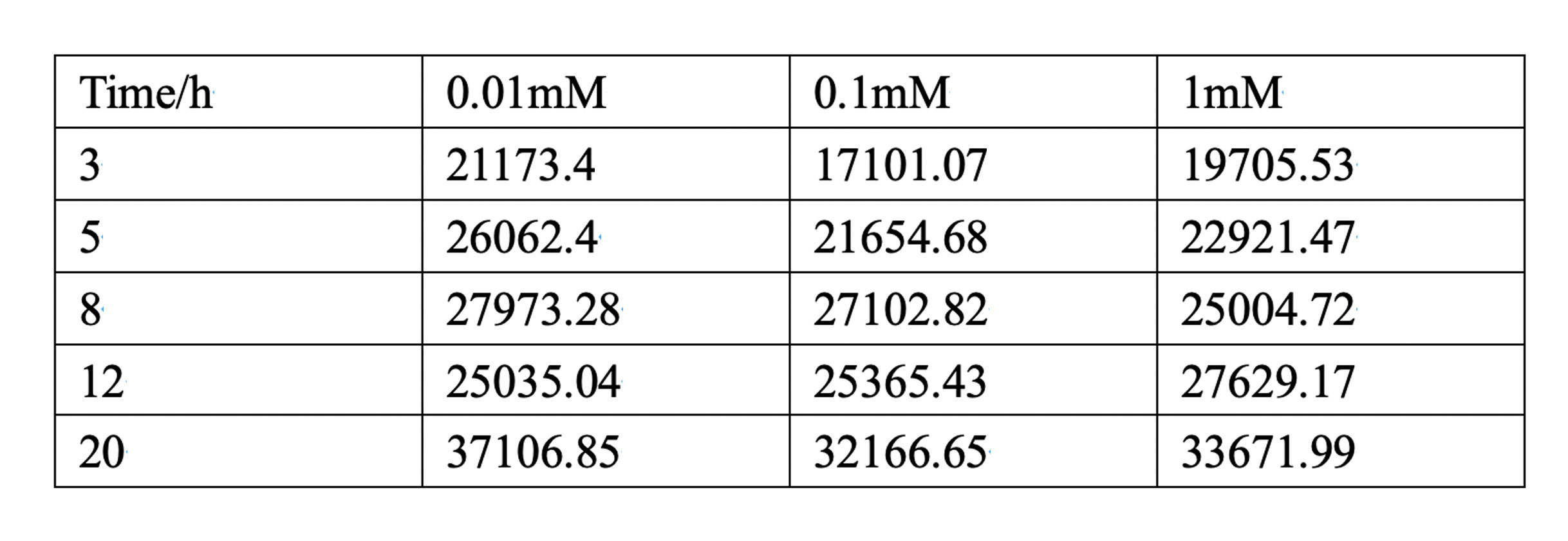In table 1, gray values were measured by Image J in order to quantify the protein expression level. In this case, we tested three concentrations of IPTG to conduct induction.
According to the scatter plots, we noticed that the cubic polynomial equation could fit the trends very well with all fitting degrees higher than 0.98.
Cubic polynomial equation: y = p1x3 + p2x2 + p3x + p4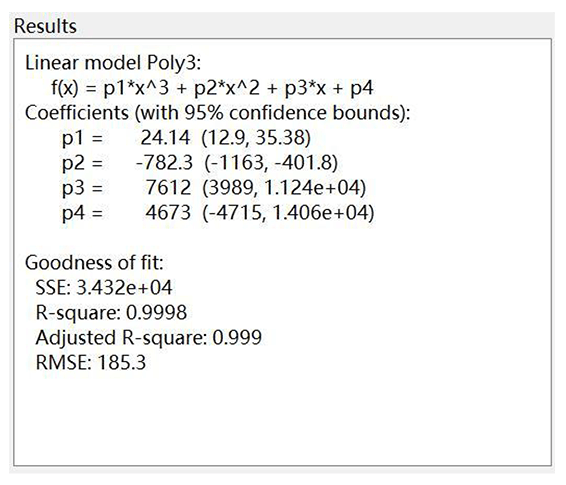Figure 1. The model result when IPTG concentration equals 0.01 mM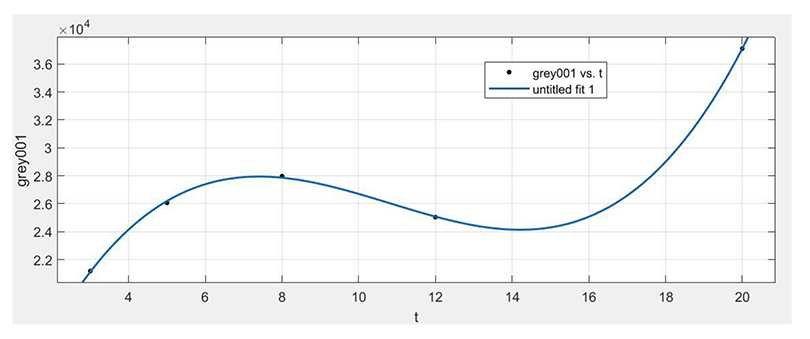Figure 2. The fitting curve of the model when IPTG concentration equals 0.01 mM
When E. coli/PKC-OP is induced by 0.01mM IPTG, it indicates that before 12 hours there is a peak at 7 hours when the protein was expressed the best. Otherwise, if there is enough time given, the protein could be expressed more after 18 hours and the induction duration to be chosen will depend on the production plan.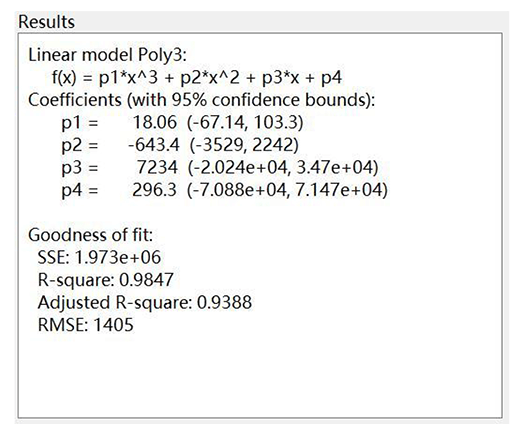Figure 3. The model result when IPTG concentration equals 0.1 mM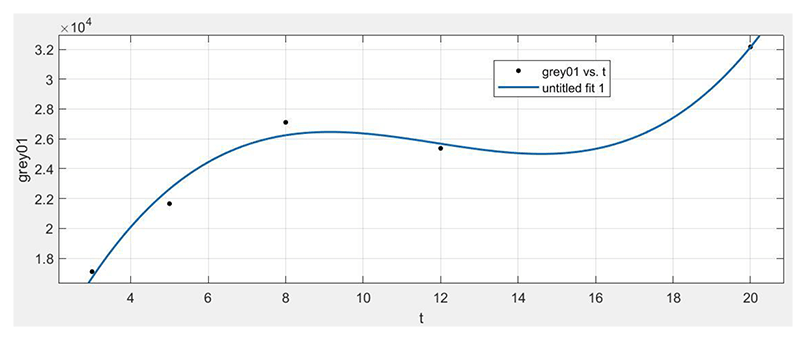Figure 4. The fitting curve of the model when IPTG concentration equals 0.1 mM
When E. coli/PKC-OP is induced by 0.1mM IPTG, we can see that the basic trend of the protein expression level is increasing as the induction time increases. In this case, we would suggest the least induction time to be 8 hours.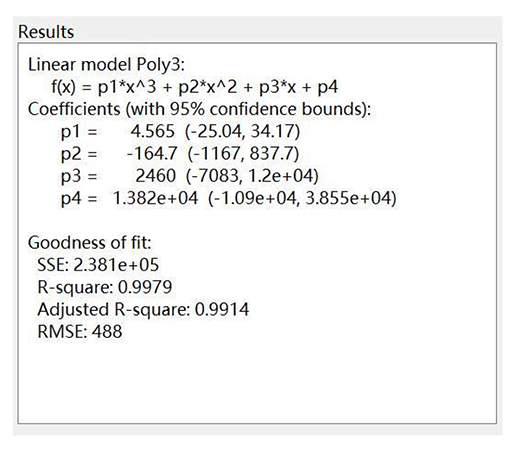Figure 5. The model result when IPTG concentration equals 1 mM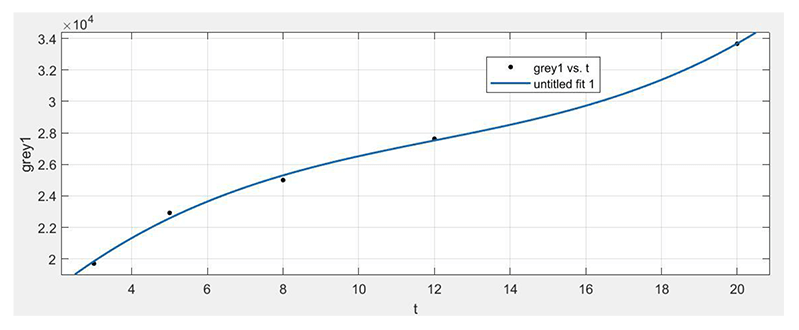Figure 6. The fitting curve of the model when IPTG concentration equals 1 mM
When E. coli/PKC-OP was induced by 1 mM IPTG, it shows a clear increasing trend of the protein expression level as the induction time increases.
Comparison
In order to further analyze the effect of the IPTG concentration on the protein expression level of E. coli/PKC-OPO, we put these three lines on the same page as shown in figure 7 and the coding is given below:

clear;clc;
t0=[3 5 8 12 20];
grey001=[21173.401 26062.4 27973.278 25035.036 37106.845];
grey01=[17101.066 21654.681 27102.823 25365.43 32166.652];
grey1=[19705.53 22921.471 25004.723 27629.166 33671.986];
p001=polyfit(t0,grey001,3);
p01=polyfit(t0,grey01,3);
p1=polyfit(t0,grey1,3);
t=[0:0.1:22];
y001=polyval(p001,t);
y01=polyval(p01,t);
y1=polyval(p1,t);
plot(t,y001,t,y01,t,y1,'LineWidth',1)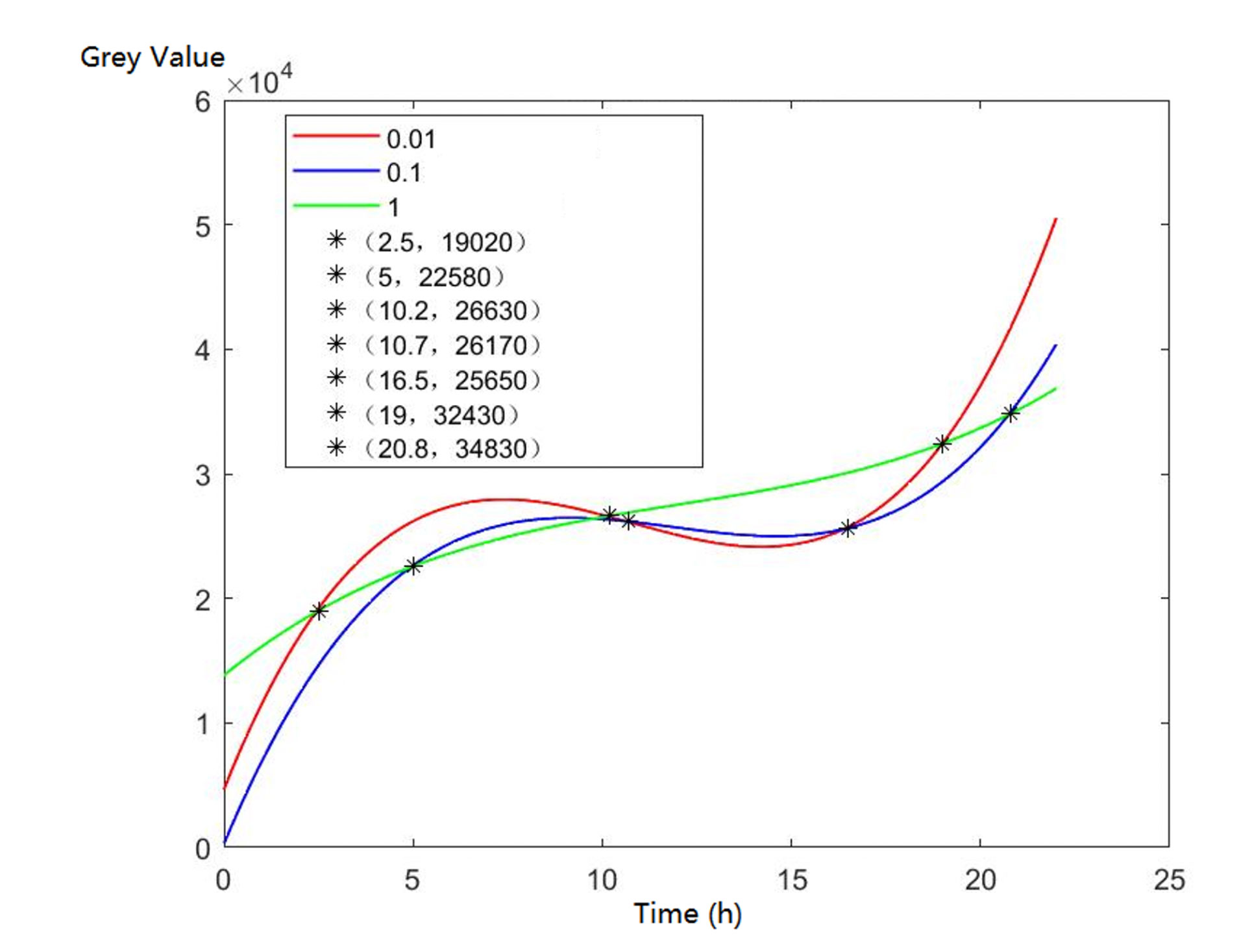Figure 7. Comparison graph of the fitting curves of three models
Based on the graph where we can see several cross points’ coordinates of lines, we could adjust the IPTG concentration according to the induction time which could be pre-decided by the production plan in the future.
When the induction time is given less than 2.5 hours, 1 mM IPTG would be more recommended;
When the induction time is given during 2.5 hours 10.2 hours, 0.01 mM IPTG would be more recommended;
When the induction time is given during 10.2 hours ~ 19 hours, 1 mM IPTG would be more recommended;
When the induction time is given very enough like more than 19 hours, 0.01 mM IPTG would be more recommended.